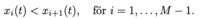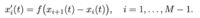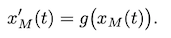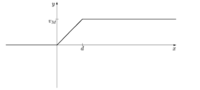# Differential equation, recursive

#### Karl Karlsson

##### New member
We will look at a model with a number of driverless cars that use the same control system. Suppose that M cars drive one after another on a road. The position of car i at time t is called xi(t). The cars are arranged so thatThe function g is just equal to g = 5 m/s for all t.The function f is f(x)=x/3, for 0<x<75 and is for 75<x equal to 25.

Let M = 10

0 < t < 40s

Let the positions of the cars at time t = 0 be given by xi (0) = d · i, i = 1, ..., M

You are supposed to implement eulers method to solve this problem:
This problem is solved with paper and pencil. If the time step h is too large, the cars may "pass each other" in the Euler solution, ie. xi^n> x(i + 1)^n (this is not x times i + 1 nor is i+1 an input for the function, this only represents the (i+1):th x) , for some time step n. Determine an upper limit for time step h such that it is certain that this will not happen.

I am having problem with this as there are no similar problems in my book

Regards Karl

#### Karl Karlsson

##### New member
Does anybody have any idea?

#### Subhotosh Khan

##### Super Moderator
Staff member
We will look at a model with a number of driverless cars that use the same control system. Suppose that M cars drive one after another on a road. The position of car i at time t is called xi(t). The cars are arranged so that

View attachment 15074

View attachment 15076

View attachment 15075

The function g is just equal to g = 5 m/s for all t.

View attachment 15077

The function f is f(x)=x/3, for 0<x<75 and is for 75<x equal to 25.

Let M = 10

0 < t < 40s

Let the positions of the cars at time t = 0 be given by xi (0) = d · i, i = 1, ..., M

You are supposed to implement eulers method to solve this problem:
This problem is solved with paper and pencil. If the time step h is too large, the cars may "pass each other" in the Euler solution, ie. xi^n> x(i + 1)^n (this is not x times i + 1 nor is i+1 an input for the function, this only represents the (i+1):th x) , for some time step n. Determine an upper limit for time step h such that it is certain that this will not happen.

I am having problem with this as there are no similar problems in my book

Regards Karl
I am not sure I understand the problem!

On a different note -

You did not respond to our tutor's request in: